# Carboxylic Acid - JEE Main Previous Year Questions with Solutions

JEE Main Previous Year Papers Questions of Chemistry with Solutions are available at eSaral. Practicing JEE Mains chapter wise questions of Chemistry will help the JEE aspirants in realizing the question pattern as well as help in analyzing weak & strong areas. Simulator Previous Years AIEEE/JEE Mains Questions
Q. In the chemical reactions,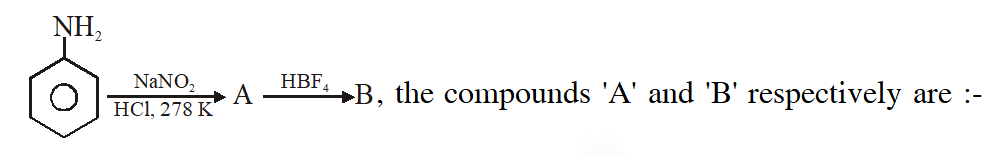(1) Nitrobenzene and chlorobenzene (2) Nitrobenzene and fluorobenzene (3) Phenol and benzene (4) Benzene diazonium chloride and fluorobenzene [AIEEE-2010]
Ans. (4)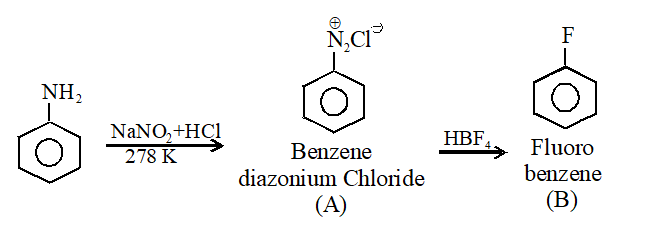Q. In the chemical reactions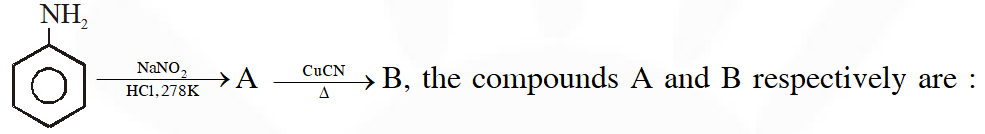(1) Fluorobenzene and phenol (2)Benzene diazonium chloride and benzonitrile (3) Nitrobenzene and chlorobenzene (4) Phenol and bromobenzene [AIEEE-2011]
Ans. (2)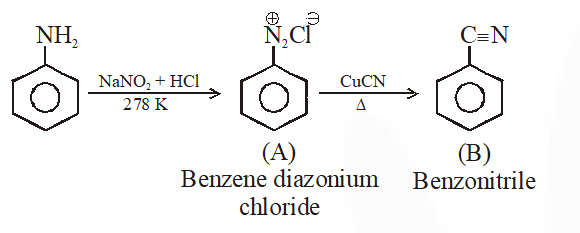Q. Compound (A), $\mathrm{C}_{8} \mathrm{H}_{9} \mathrm{Br}$, gives a white precipitate when warmed with alcoholic $\mathrm{AgNO}_{3}$. Oxidation of (A) gives an acid (B), $\mathrm{C}_{8} \mathrm{H}_{6} \mathrm{O}_{4}$. (B) easily forms anhydride on heating. Identify the compound (A):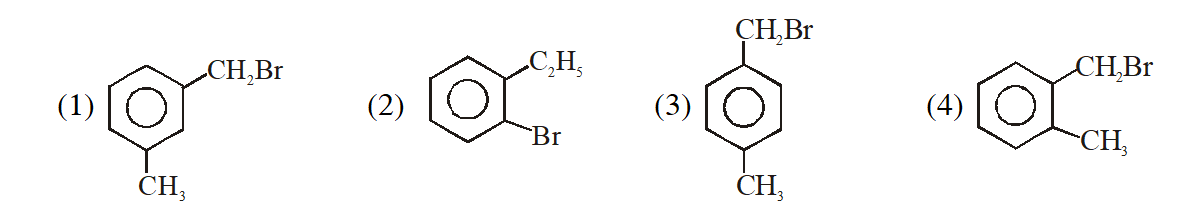[AIEEE-2013]
Ans. (4)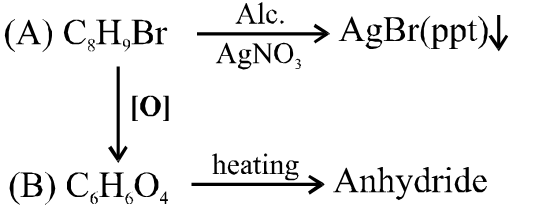since B on heating gives anhydride so B is 1,2 dicarboxylic acid. It contains two Benzylic carbon at 1,2 position so on oxidation it produces 1, 2-dicarboxylic acid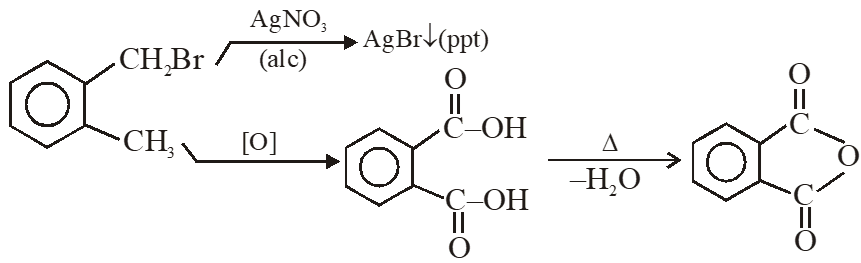Q. An organic compound A upon reacting with $\mathrm{NH}_{3}$ gives B. On heating, B gives C. C in presence of KOH reacts with $\mathbf{B} \mathbf{r}_{2}$ to give $\mathrm{CH}_{3} \mathrm{CH}_{2} \mathrm{NH}_{2}$. A is :- (1) $\mathrm{CH}_{3} \mathrm{COOH}$ (2) $\mathrm{CH}_{3} \mathrm{CH}_{2} \mathrm{CH}_{2} \mathrm{COOH}$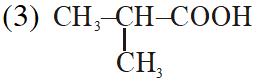(4) $\mathrm{CH}_{3} \mathrm{CH}_{2} \mathrm{COOH}$ [AIEEE-2013]
Ans. (4)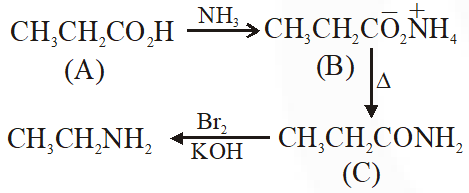Q. On heating an aliphatic primary amine with chloroform & ethenolic potassium hydroside the organic compound formed is (1) An alkyl cyanide (2) An alkyl isocyanide (3) an alkanol (4) an alkanediol [AIEEE-2014]
Ans. (2) It is a carbyl amine reaction used for identification of primary amine also known as isocynide test because of offensive smell of isocynide.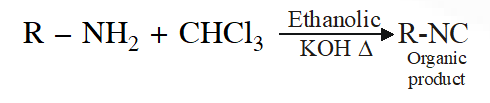Q. In the reaction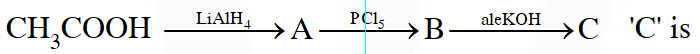(1) Ethylene (2) Acetyl chloride (3) Acetaldehyde (4) Acetylene. [AIEEE-2014]
Ans. (1)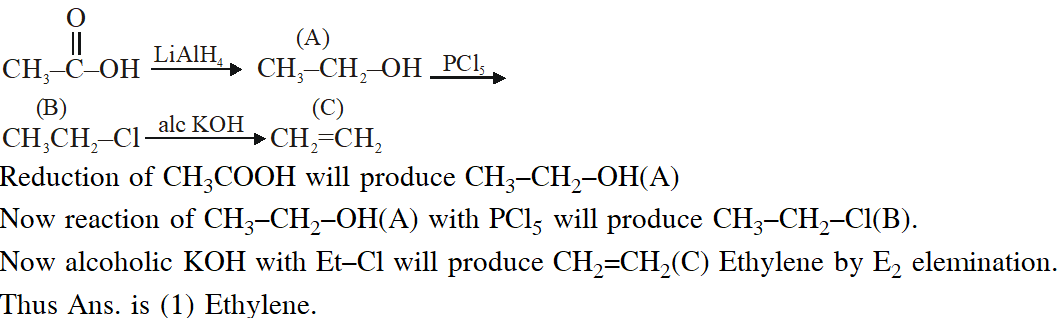Q. In the presence of a small amount of phosphorous, aliphatic carboxylic acids react with chlorine or bromine to yield a compound in which  - hydrogen has been replaced by halogen. This reaction is known as : (1) Etard reaction (2) Hell - Volhard - Zelinsky reaction (3) Wolff - Kischner reaction (4) Rosenmund reaction [JEE(Main)-2015]
Ans. (2)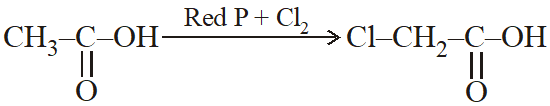Q. In the Hofmann bromamide degradation reaction, the number of moles of NaOH and $\mathrm{Br}_{2}$ used per mole of amine produced are :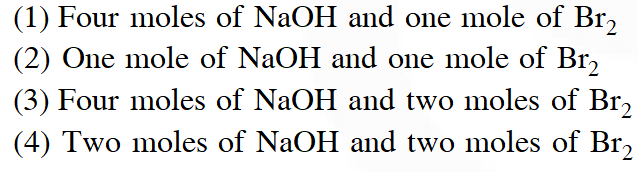[JEE(Main)-2016]
Ans. (1)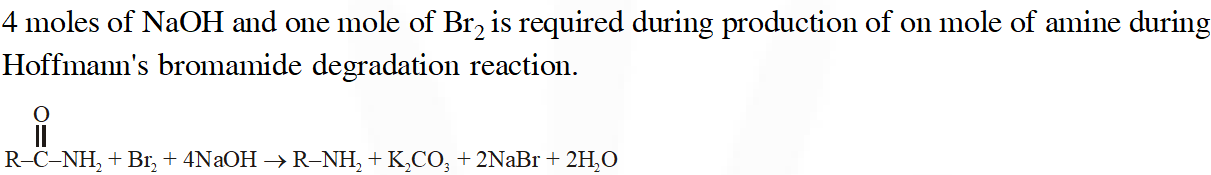Q. The major product obtained in the following reaction is :-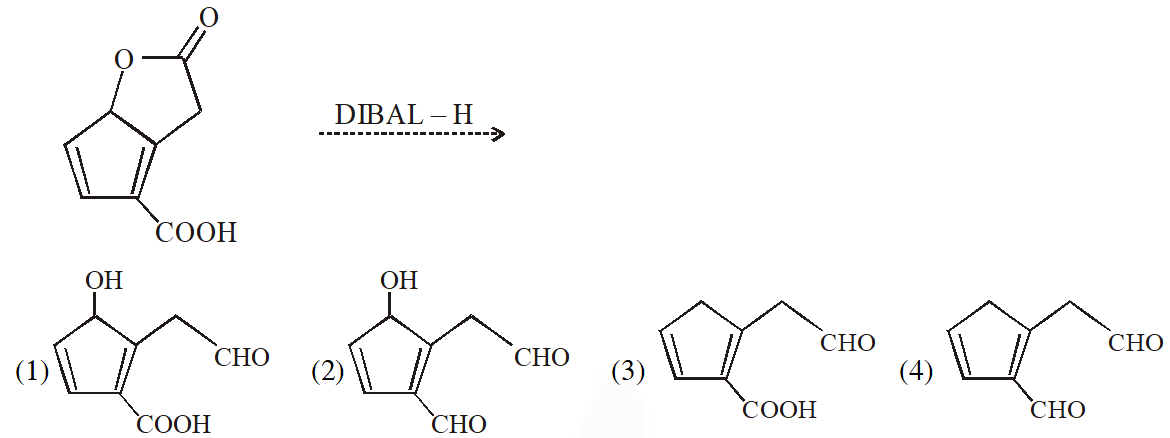[JEE - Main 2017]
Ans. (1) DIBAL – H is electrophilic reducing agent reduces cynide, esters, lactone, amide, carboxylic acid into corresponding Aldehyde (partial reduction)
Q. Sodium salt of an organic acid 'X' produces effervescence with conc. $\mathrm{H}_{2} \mathrm{SO}_{4}$. 'X' reacts with the acidified aqueous CaCl2 solution to give a white precipitate which decolourises acidic solution of $\mathrm{KMnO}_{4}$. 'X' is :- (1) $\mathrm{C}_{6} \mathrm{H}_{5} \mathrm{COONa}$ (2) HCOONa (3) CH3COONa (4) $\mathrm{Na}_{2} \mathrm{C}_{2} \mathrm{O}_{4}$ [JEE - Main - 2017]
Ans. (4)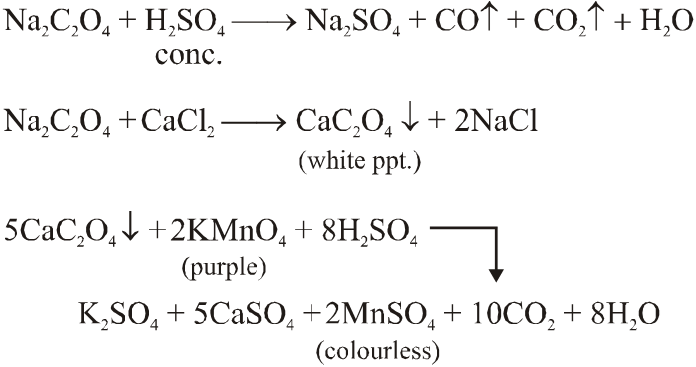Q. Which of the following compounds will be suitable for Kjeldahl's method for nitrogen estimation ?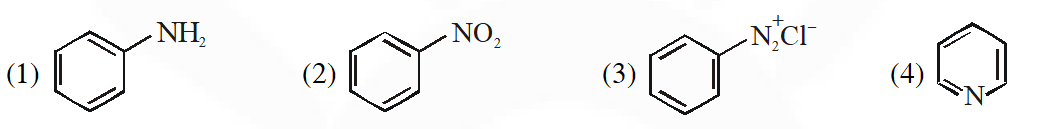[JEE(Main)-2018]
Ans. (1) Kjeldahl's is suitable for Aniline. This method is used for quantitative analysis of N compound in organic substance $\left(\mathrm{NH}_{3} / \mathrm{NH}_{4}^{+}\right) .$